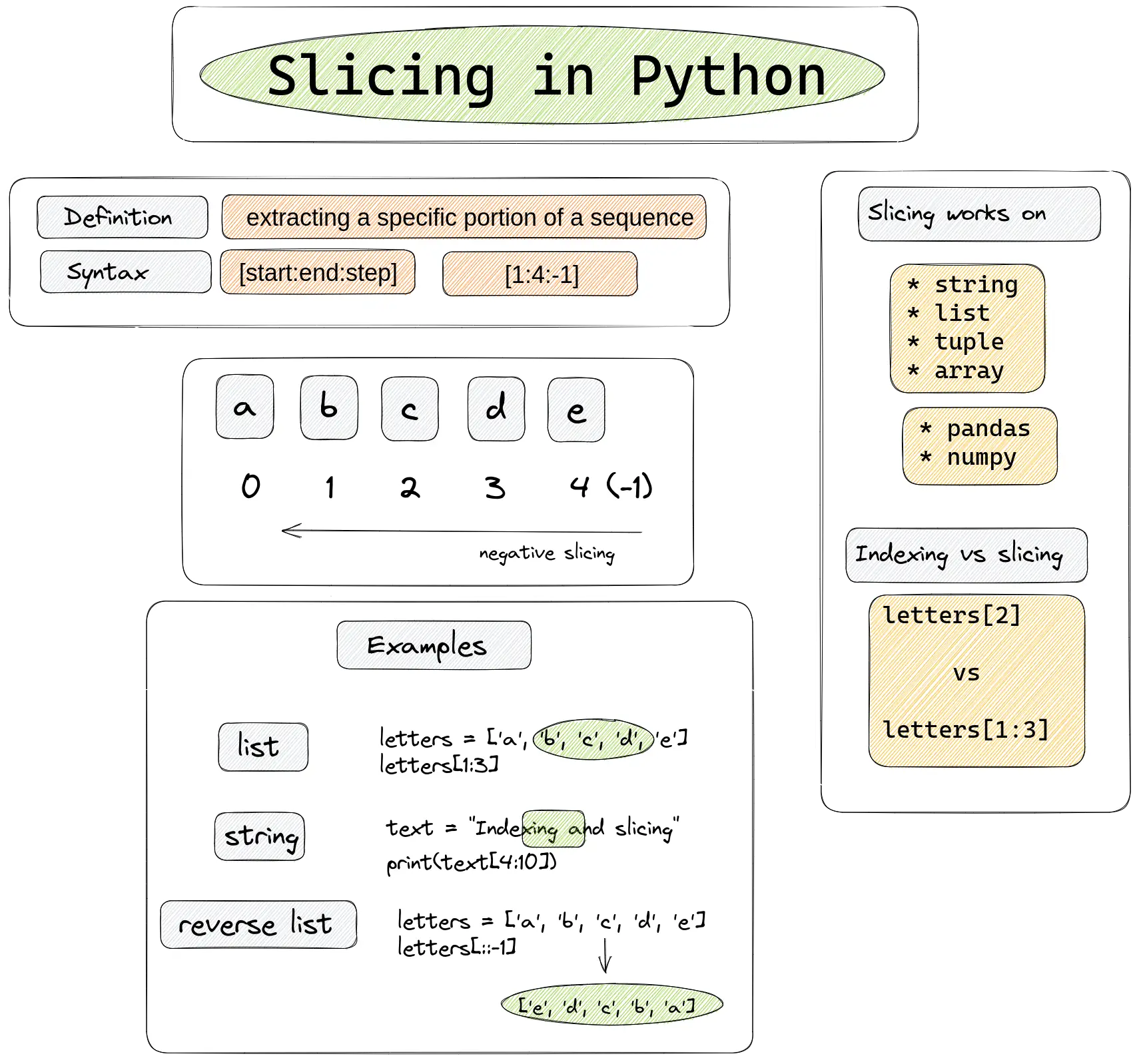Slicing in Python is a powerful feature that allows easy extraction and modification of parts of sequences. For example extraction of sublists from another list or last N characters from a string.

Slicing in Python, refers to the process of extracting a specific portion of a sequence, such as:

• string
• list
• tuple
• array

Slicing can be done using the slice notation, which usually consists of two indices separated by a colon - `:`. You can find more about the slicing syntax in the next section.## Slicing syntax

The slice notation `[start:end:step]` is used to extract a portion of a sequence, where:

• the start index is the first element you want to include
• the end index is the first element you want to exclude
• the step is the number of elements to skip between each item in the slice

Start and end indices are optional. The same is for the step.

In the case of `[::-1]`:

• the start and end indices are not specified, meaning they take the default values of 0 and -1 or `len(sequence)`
• the step is -1, so it will take every element in the sequence in reverse order

## Indexing and slicing

Indexing and slicing are ways to access specific elements or portions of a sequence.

Indexing is used to access a single element of a sequence by its position, or index. The index of the:

• first element is 0
• the second element is 1 and so on.
• the last element can be accessed by -1 or `len(sequence)`

To access an element at a specific index, you can use the square brackets notation, like this:

``````letters = ['a', 'b', 'c', 'd', 'e']
print(letters)
``````

result:

``````c
``````

Slicing is used to access a range of elements from a sequence:

``````letters = ['a', 'b', 'c', 'd', 'e']
letters[1:3]
letters[::2]
``````

result:

``````['b', 'c']
['a', 'c', 'e']
``````

## Negative slicing

We can also use negative indexes in slicing, which starts counting from the end of the sequence. For example :

``````letters = ['a', 'b', 'c', 'd', 'e']
letters[-3:-1]
``````

result into:

``````['c', 'd']
``````

while:

``````letters[1:3]
``````

result into:

``````['b', 'c']
``````

## What is [::1] in Python?

In Python `[::1]` or `[::-1]` is used to extract every element from a sequence, in the same or reverse order.

`[::1]` is equivalent to slicing with just one colon - `[:]`.

### slicing with positive step

Slicing with a positive step is done from left to right. By default step is 1:

``````letters = ['a', 'b', 'c', 'd', 'e']
print(letters[::1])
``````

result:

``````['a', 'b', 'c', 'd', 'e']
``````

### reverse order slicing

Negative step change the order of slicing from right to left:

``````letters = ['a', 'b', 'c', 'd', 'e']
print(letters[::-1])
``````

result:

``````['e', 'd', 'c', 'b', 'a']
``````

Next we will cover several examples for slicing in Python.

## Slicing list

Extracting a specific range of elements from a Python list:

``````numbers = ['a', 'b', 'c', 'd', 'e']
print(numbers[2:4])
``````

Extracted sublist is:

``````['c', 'd']
``````

## Modify list with slicing

We can even modify Python list by using slicing:

``````letters = ['a', 'b', 'c', 'd', 'e']
letters[1:3] = ['x', 'y']
print(letters)
``````

The result is a list with changed elements:

``````['a', 'x', 'y', 'd', 'e']
``````

## Slicing string

Extracting a specific range of characters from a string or getting a substring:

``````text = "Indexing and slicing"
print(text[4:10])
``````

We get this substring by slicing:

``````xing a
``````

## Python slice array

To extract a specific range of rows and columns from a 2D array we can use slicing. Let's have the following array in Python:

``````import numpy as np
matrix = np.array([[1, 2, 3], [4, 5, 6], [7, 8, 9], [10, 11, 12]])
print(matrix)
``````
``````[[ 1  2  3]
[ 4  5  6]
[ 7  8  9]
[10 11 12]]
``````

we can use slicing to extract subarray:

``````print(matrix[0:2, 1:3])
``````

which results into:

``````[[2 3]
[5 6]]
``````

## Slicing tuple

Extracting the elements of a tuple:

``````letters = ('a', 'b', 'c', 'd', 'e')
print(letters[-2:])
``````

extracting last two elements of a tuple:

``````('d', 'e')
``````

## Slice every n-th element

We can use slicing to extract every n-th element from a sequence. For example extracting every second element:

``````letters = ['a', 'b', 'c', 'd', 'e']
letters[::2]
``````

result:

``````['a', 'c', 'e']
``````

## Slicing in Pandas

Slicing is used in many libraries like numpy, pandas, etc.

Slicing in Pandas is similar to Python slicing for sequences, but it is applied to DataFrame and Series.

Pandas offers a variety of ways to slice and index data, including:

• label-based indexing using the `.loc` and `.at` attribute
• select data by the label of the rows and columns
• position-based indexing using the `.iloc` and `.iat` attribute
• select data by the integer position of the rows and columns
• Boolean indexing
• select data based on a boolean condition.

Examples of slicing in Pandas:

select all rows and columns 'A' to 'C'

``````df.loc[:, 'A':'C']
df.iloc[3:5, 0:2] # select rows 3 to 4 and columns 0 to 1
``````

select rows 0 to 2 and columns 1 to 3

``````df.iloc[0:3, 1:4]
``````